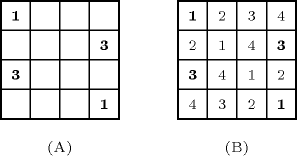### 3.7.133. Latin square

##### Figure 3.7.36. A partially filled Latin square and a possible completionA constraint that can be used for modelling the Latin square completion problem. A Latin square of order $n$ is an $n×n$ array in which $n$ distinct numbers in $\left[1,n\right]$ are arranged so that each number occurs once in each row and column. The Latin square completion problem is to complete a partially filled Latin square. Part (A) of Figure 3.7.36 gives a partially filled Latin square, while part (B) provides a possible completion. The Latin square completion problem is a pattern that occurs in some applications such that dynamic wavelength routing or sport timetabling.